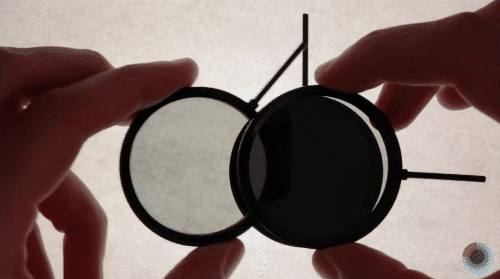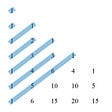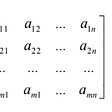# Wait, Change is Better Than Quantity?

## An Introduction to Differential Equations

When we think about the math that we have learned throughout school, the overwhelming majority deals with absolute quantities. For example, if given the linear function f(x) = x + 2, for whatever input x, we obtain an output that is a fixed number quantity: if x = 2, f(x) = 4, and if x = 5.5, f(x) = 7.5. For the longest time, I thought that this was all that math entailed. That was until I first learned about calculus.

In calculus, the derivative was introduced, opening up the world of representing rate of change. I was surprised to see how useful this rate of change was: it could tell us where the function was increasing, decreasing, or if it was at a maximum or minimum. However, as we moved to differential equations, I became the most intrigued. At the heart of it, differential equations equate a function to its derivative. Instead of f(x) = … we have dy/dx = … Differential equations are very important in the world of modeling, as multiple laws from physics, economics, and chemistry can be represented by these types of equations. The heat equation in physics and the Black-Scholes equation in finance are just a few famous models.

In this article, I’ll start with a brief introduction to differential equations, including methods for separable differential equations and first order linear differential equations.

# Separable Differential Equations

As mentioned before, differential equations talk in terms of change rather than quantity. Therefore, when we solve a differential equation, our answer is in terms of a function. In fact, there are infinitely many answers to basic differential equations, as we are simply finding an antiderivative in most cases. The first type of differential equation we’ll consider are Separable Differential Equations.

Consider the problem below.

We are given information about the derivative of the function y, and we need to find the original function. How should we go about solving this? The name “Separable Differential Equation” should give us a subtle hint about our preferred method of tackling this problem: separate the equation such that all y’s are on one side and all x’s are on the other. We can do this by multiplying y on both sides and then multiplying dx on both sides.

Now that all of the variables and their respective differentials are set in place, we can simply integrate both sides of the equation. Doing so will give us a variation of the function y, which we can manipulate to give us our final answer.

After integration with respect to y and x on both sides, we multiply both sides of the equation by 2. Note that technically the +C would become +2C, but this simply merges back into a new constant of integration. After that, we take the positive and negative square root of both sides of the equation, and we obtain our final answer.

## First Order Linear Differential Equations

First order linear differential equations are differential equations with the following form.

If you take some time to look at this equation, you’ll notice that it is impossible to separate, meaning we’ll need to resort to some different methods. First, let’s take a look at a simple example.

Notice that this is simply a first order linear differential equation that has been modified, with P(x) = 1/x and Q(x) = 5; we just multiplied both sides of the equation by x. Now, the left side of the equation simply can be shortened to the “product rule” of xy.

From there, simply integrate both sides of the equation and solve for y. The logic here is actually extremely beautiful and smooth: in order to solve first order linear differential equations, we should try and model the form of the product rule and then integrate.

## General Method

However, not all problems will be as simple as the previous example. In most cases, we’ll need to manipulate the equation by multiplying the Integrating Factor.

The integrating factor is always e to the power of the integral of P(x). Intuitively, this makes sense because when we multiply the equation by this function, product rule comes into play. Note that if there are any x’s in front of the dy/dx, modify the equation to make it so that dy/dx is left alone. To get a clearer sense, let’s explore the example problem above.

After we multiply by the integrating factor, the left side of the equation becomes the “product rule” of y and the integrating factor itself (note that if we differentiate the product of the two, due to the chain rule, we’ll get the left-hand side of the equation). Integrating both sides and then isolating y, we solve our differential equation.

## Wrapping Up

Differential equations are huge in the scene of mathematics, as they can model real-world scenarios and phenomena. The level of difficulty associated with some specific differential equations are extremely high, yet humans can still understand certain characteristics of their solutions. I presented two basic types of differential equations that provide a nice segue into learning more about the topic. I thoroughly enjoyed learning about them both in junior and senior year of high school, and I hope you can appreciate the beauty of the math as well!

--

--

--

## More from Albert Ming

18 || High School Senior || Stats & Math

Love podcasts or audiobooks? Learn on the go with our new app.

## Clarifying Entropy## About Me — Albert Ming## BrainCycle: Working Out Elliptic Curves with Elliptical Cardio## A proof that superpositions collapse | Summary of 3b1b video “Some light quantum mechanics”## The Fibonacci Sequence and The Powers of Two## What is the Difference Between Speed and Velocity? — The Prodigious## My Linear Algebra Journey Begins Now## Albert Ming

18 || High School Senior || Stats & Math

## Simple Pigeonhole principle, but interesting result — Journey in Combinatorics## Pigeonhole Principle and Functions## Matrix & Matrix Operations | Numerical Linear Algebra | Part 1## Part 2: Vectors